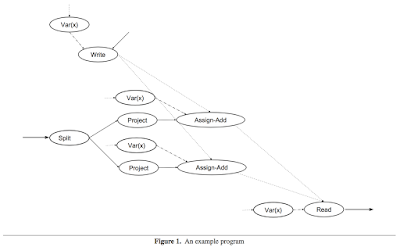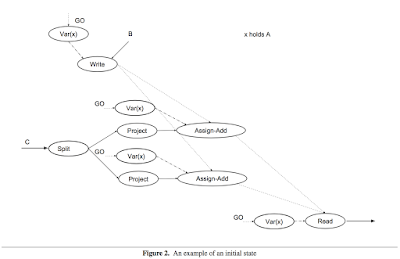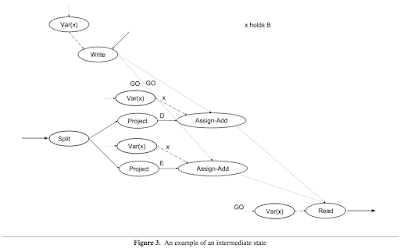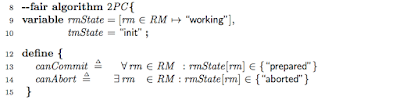## Tuesday, November 21, 2017

### Paper summary: A Computational Model for TensorFlow

This paper appeared in MAPL 17. It is written by Martin Abadi, Michael Isard, and Derek G. Murray at Google Brain. It is a 7-page paper, and the meat of the paper is in Section 3.

I am interested in the paper because it talks about TLA+ modeling of TensorFlow graphs and uses that for creating an operational semantics for TensorFlow programs. In other words, the paper provides a conceptual framework for understanding the behavior of TensorFlow models during training and inference.

As you recall, TensorFlow relies on dataflow graphs with mutable state. This paper describes a simple and elementary semantics for these dataflow graphs using TLA+. The semantics model does not aim to account for implementation choices: it defines what outputs may be produced, without saying exactly how. A framework of this kind does not just have theoretical/academical value; it can be useful to assess correctness of TensorFlow's dataflow graph (symbolic computation graph) rewriting optimizations such as those in XLA.

## TensorFlow refresher

In TensorFlow, dataflow graphs support both training and inference. That is, a computation may perform one or more steps of training for a machine-learning model, or it may be the application of a trained model. TensorFlow models are assembled from primitive operations by function composition. The operations are implemented by kernels that can be run on particular types of devices (for instance, CPUs or GPUs).

In addition to edges for communicating tensors, a graph may include control edges that constrain the order of execution. This order can affect performance as well as the observable semantics in the presence of mutable state.

A client typically constructs a graph using a front-end language such as Python. Then the client can make a call to run the graph, specifying which inputs to "feed" and which outputs to "fetch". TensorFlow propagates the input values, repeatedly applying the operations prescribed by the graph, until no more nodes can fire. The order in which nodes fire is constrained by data dependencies and control edges, but is not necessarily unique. The execution ends with values on the graph's output edges. Often, a graph is executed multiple times. Most tensors do not survive past a single execution of the graph. However, mutable state does persist across executions.

## Core Computational Model

This section presents TLA+ modeling of the TensorFlow.

TensorFlow values include tensors and variables. The TLA+ model distinguishes three kinds of edges: tensor edges, variable edges, and control edges.

Operations are of several kinds: Functions, Var(x), Read, Assign. A TensorFlow program consists of a directed acyclic graph G, plus a mapping (a "labelling") L from nodes of G to TensorFlow operations.A TensorFlow program starts with non-EMPTY input edges, consumes the values on those edges, and repeatedly propagates them, applying operations, until no more nodes can fire. In the course of such an execution, each node fires exactly once, and the execution ends with non-EMPTY output edges. The order in which nodes fire is not necessarily unique: determinism is not always expected or desired. For example, lock-free stochastic gradient descent is a common source of intentional race conditions.

Each change of state in the behavior is caused by the execution (i.e., the firing) of exactly one node in the graph. A condition for whether a node n can cause a change from a state s to a state s′ is that for all its incoming control edges d, InTransit(d) = GO in s and InTransit(d) = EMPTY in s′, and for all its outgoing control edges e, InTransit(e) = GO in s′. Moreover, InTransit(d) must be the same in s and in s′ for all edges d not incident on n, and VarValue(x) must be the same in s and in s′ for all variables x, except in the case where L(n) = Assign-f for some function f.Figure 3 shows a computation that *assign-adds* x:=x+D and x:=x+E. The *assign-adds* commute for x, but *writes* alone do not commute and leads to a
last-write-wins race condition, as in Figure 4.A race condition can be avoided by other means, such as implementing mutexes using TensorFlow control primitives.

## Further work

The paper claims that this semantics can be generalized to accommodate cyclic execution graphs in TensorFlow. In that generalization, instead of firing exactly once, each node fires at most once in each "execution context", where an "execution context" identifies an iteration of each (possibly nested) loop that contains the node.